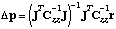# Manual Page for Command >>> GAUSS-NEWTON

Syntax
>>> GAUSS-NEWTON

Parent Command
>> OPTION

Subcommand
-

Description
This command performs Gauss-Newton steps to minimize the objective function. The Gauss-Newton algorithm assumes linearity and can be described as follows:Gauss-Newton steps are efficient if the model is linear (only one iteration required to find minimum) or nearly-linear. If the model is highly nonlinear, Gauss-Newton steps are usually too large, leading to an inefficient or even unsuccessful step. By default, iTOUGH2 uses the Levenberg-Marquardt minimization algorithm, which is a modification of the Gauss-Newton algorithm.

Example
> COMPUTATION
>> OPTION
>>> use GAUSS-NEWTON minimization algorithm
<<<
>> STOP
after >>> :1 ITERATION
<<<
<<